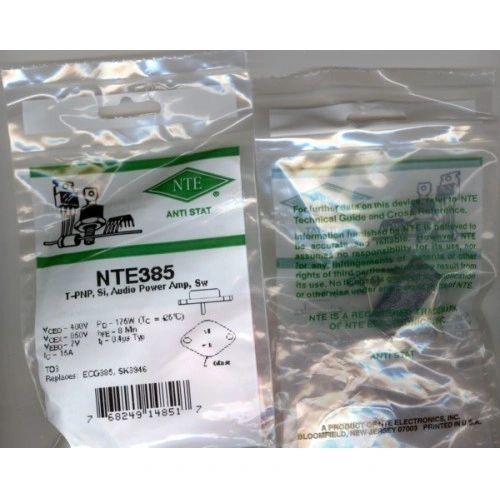•# TRANSISTOR PNP NTE 385 ELECTRONICS PARTS

\$18.00 \$10.00
Free Shipping!

TRANSISTOR

NTE 385

The NTE385 is a silicon NPN transistor in a TO3 type package designed high voltage, high speed, power switching in inductive circuits where fall time is critical. This device is particularly suited for line operated switchmode applications.

Features:

• Fast Turn-Off Times
Absolute Maximum Ratings:

Collector-Emitter Voltage, VCEO(sus) 400V Collector-Emitter Voltage (VBE = -1.5V), VCEX 850V Emitter-Base Voltage, VEB 7V Collector Current, IC
Continuous
Peak (Note 1)
15A
30A
60A
Base Current, IB
Continuous
Peak (Note 1)
5A
20A
Total Power Dissipation (TC = +25?C), PD 175W Derate above 25?C 1.0W/?C Total Power Dissipation (TC = +100?C), PD 100W Operating Junction Temperature Range, TJ -65? to +200?C Storage Temperature Range, Tstg -65? to +200?C Maximum Thermal Resistance, Junction-to-Case, RthJC 1.0?C/W Lead Temperature (During Soldering, 1/8" from case, 5sec), TL +275?C

Note 1. Pulse test: Pulse Width = 5ms, Duty Cycle

Electrical Characteristics: (TC = +25?C unless otherwise specified)

Parameter Symbol Test Conditions Min Typ Max Unit OFF Characteristics (Note 2) Collector-Emitter Sustaining Voltage VCEO(sus) IC = 200mA, IB = 0, L = 25mH 400 - - V Collector Cutoff Current ICEX VCEX = 850V, VBE(off) = 1.5V - - 0.2 mA VCEX = 850V, VBE(off) = 1.5V, TC = +125?C - - 2.5 mA ICER VCE = 850V, RBE = 10 Ohm - - 0.5 mA VCE = 850V, RBE = 10 Ohm, TC = +100?C - - 3.0 mA Emitter Cutoff Current IEBO VBE = 5V, IC = 0 - - 0.1 mA Emitter-Base Breakdown Voltage V(BR)EBO IE = 50mA, -IC = 0 7 - - V ON Characteristics (Note 2) DC Current Gain hFE VCE = 5V, IC = 10A 8 - - Collector-Emitter Saturation Voltage VCE(sat) IC = 10A, IB = 2A - - 1.5 V IC = 10A, IB = 2A, TC = +100?C - - 2.0 V IC = 8A, IB = 1.6A - - 1.5 V IC = 8A, IB = 1.6A, TC = +100?C - - 2.0 V Base-Emitter Saturation Voltage VBE(sat) IC = 10A, IB = 2A - - 1.6 V IC = 10A, IB = 2A, TC = +100?C - - 1.6 V Dynamic Characteristics Output Capacitance Cob VCB = 10V, IE = 0, f = 1kHz - - 350 pF Switching Characteristics (Resistive Load) Delay Time td VCC = 300V, IC = 10A, IB = 2A, tp = 30?s, Duty Cycle BE(off) = 5V - 0.1 0.2 ?s Rise Time tr - 0.4 0.7 ?s Storage Time ts - 1.3 2.0 ?s Fall Time tf - 0.2 0.4 ?s Switching Characteristics (Inductive Load, Clamped) Storage Time tsv IC = 10A, IB1 = 2A, TC = +25?C - 1.3 - ?s Fall Time tfi - 0.06 - ?s Storage Time tsv IC = 10A, IB1 = 2A, TC = +100?C - 1.5 2.5 ?s Crossover Time tc - 0.3 0.6 ?s Fall Time tfi - 0.17 0.35 ?s

Note 2. Pulse test: Pulse Width = 300?s. Duty Cycle cl = 300V, VBE(off) = 5V, LC = 180?H.

THIS SALE IS FOR 1 EACH

NOTE:

ALL ELECTRONICS ARE UNTESTED

SOLD AS IS